# Standard Can Size Measurements

• Picnic = 10-1/2 to 12 oz. = 1-1/4 cups
• 12 oz. vacuum = 12 oz. = 1-1/2 cups
• #1 can = 11 oz. = 1-1/3 cup
• #1 tall can = 16 oz. = 2 cups
• #1 square can = 16 oz. = 2 cups

• #2 can = 1 b. 4 oz. or 1 pint 2 fluid oz. = 2-1/2 cups
• #2-1/2 can = 1 lb. 13 oz. = 3-1/2 cups
• #2-1/2 square can = 31 oz. = scant 4 cups
• #3 can = 4 cups
• #3 squat can = 2-3/4 cups
• #5 can = 7-1/3 cups
• #10 can = 13 cups
• #300 can= 14 to 16 oz. = 1-3/4 cups
• #303 can = 16 to 17 oz. = 2 cups
• Baby food jar = 3-1/2 to 8 oz. depends on size
• Condensed milk = 15 oz. = 1-1/3 cups
• Evaporated milk = 6 oz. = 2/3 cup
• Evaporated milk = 14-1/2 oz. = 1-2/3 cups
• Frozen juice concentrate = 6 oz. = 3/4 cup

By Kay from Boonville, NC

Categories
Pages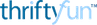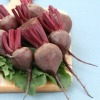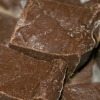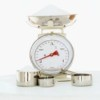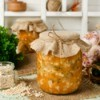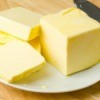More
Categories